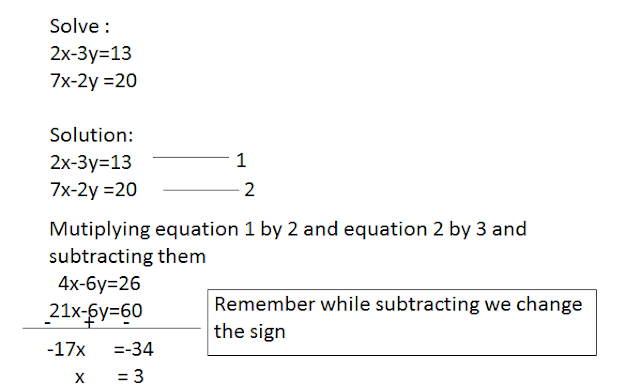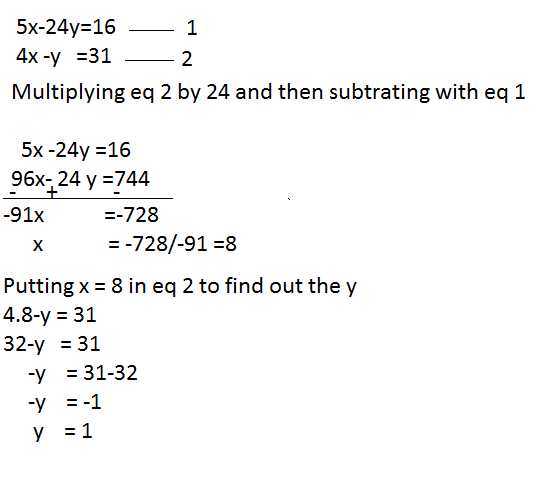# Linear Equations in Two Variables

An equation of the form ax + by + c = 0, where a,b,c ϵ R , a≠0, b≠0 and x,y are variables is called a linear variable s or an equation of first degree in two variables.

Some examples of linear equations in two variables are:
3x + 7y =9       ,           2x – y = 0

5/2 u - 9/13 v + 2 =0 , √3 t + (√5)/2 z = 7

Where x ,y; t and z are variables.

### SOLUTION OF LINEAR EQUATION IN TWO VARIABLES

A pair of value of x and y which satisfies the equation ax + by + c = 0, where a, b, c ϵ R , a≠0, b≠0 is called the solution of ax + by + c = 0.

Example: the equation 2x + y + 4

Solution:
Express y in terms of x
Put any value of x and find the corresponding value of y
The values of x and y so obtained give solution of the equation
Here 2x + y = 4
Y = 4 – 2x
Put x =0, then y = 0; x=0 and y = 4 or the ordered pair (0,4) is the solution of the given equation.

Next, put x=1, then y = 4 –(2×1) =4 – 2 =2; thus x = 1 and y = 2 or the ordered pair (1,2) is another solution of the given equation.

Let’s observe some example:
Solve: 7x + 2y =23
X – Y=2
Solution: the given equations are
7x +2y = 23 …………….. (i)
X –y = 2 …………….. (ii)
From (ii), we get  y = x – 2 ………………… (iii)
Putting (iii) in (i), we have
7x + 2(x-2) = 23
Or 7x + 2x – 4 = 23
Or 9x = 23 + 4 =27
Therefore, x = 27/9 = 3 ………………. (iv)
Putting (iv) in (ii), we have
3 –y = 2
3 – 2 = y
Y = 1
Therefore, x =3, y = 1 is the required solution.

### Solve the system of homogeneous linear equations.

Q1.   2x – 5y = 0  and  19x + y = 0### Q2. Solve 2x-3y =13 and 7x-2y =20### Q3. Solve :

5x-24y =16
4x-y     =31